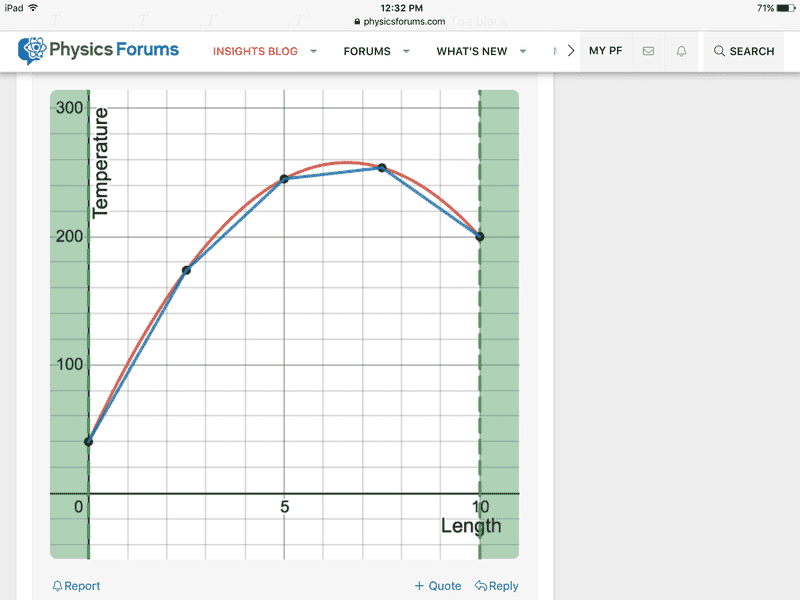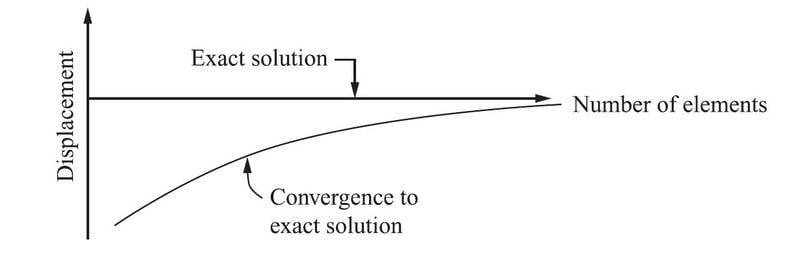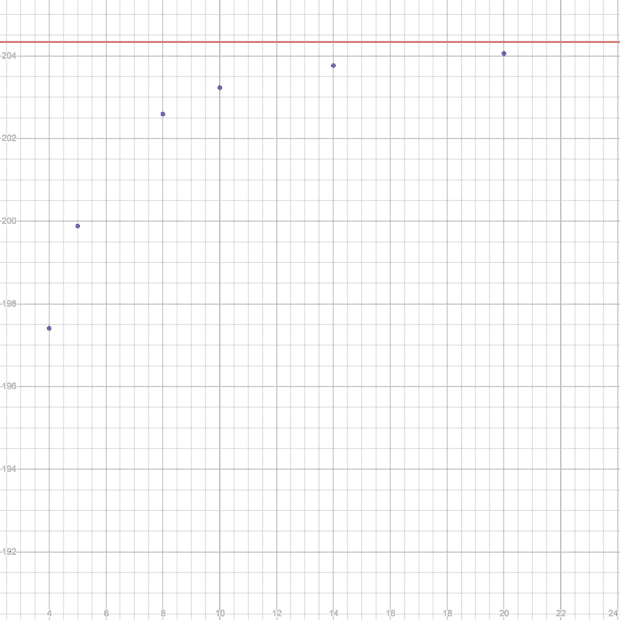# The stiffness of an FEA model depends on the mesh resolution?

feynman1
As is well known, the stiffness of an FEA model decreases with a refined mesh. What's the rigorous derivation of this?

Homework Helper
2022 Award
Why not construct the stiffness matrix for $$\frac{d^2u}{dx^2} = -f(x)$$ for $x \in (0,1)$ using elements $[nh, h(n+1)]$ with basis functions $$\begin{split} f_0 : [0,h] \to \mathbb{R} : x &\mapsto 1 - \frac{x}{h} \\ f_1 : [0,h] \to \mathbb{R} : x &\mapsto \frac{x}{h} \end{split}$$ and see what happens as you reduce $h$?

Last edited:
•feynman1
Arjan82
As is well known, the stiffness of an FEA model decreases with a refined mesh. What's the rigorous derivation of this?
Euhrm... sources?

Considering that the stiffness of the construction decreasing with mesh refinement would be really silly, do you mean the stiffness of the differential equation? But even in this case I'm not sure about this, why would this be true in general? So again: sources?

Homework Helper
2022 Award
Instead of an admonishment I will provide a tangential and perhaps incorrect annswer.
I recommend Galileo Galilei "Two New Sciences" where one of which is scale invariance and the strength of materials . To my mind these arguments point directly quantum mechanics which set the scale by which big and small are tto be measured
I believe the examination of FEA issues withion scale of the simulation are really a recapitulation of Galileo's arguments about his brave new world. Read the original!

.

Last edited:
•berkeman
Arjan82
Instead of an admonishment I will provide a tangential and perhaps incorrect annswer.
I recommend Galileo Galilei "Two New Sciences" where one of which is scale invariance and the strength of materials . To my mind these arguments point directly quantum mechanics which set the scale by which big and small are tto be measured
I believe the examination of FMEA issues withion scale of the simulation are really a recapitulation of Galileo's arguments about his brave new world. Read the original!

.

This answer and the relation to the OP's question is completely lost to me...

Arjan82
Homework Helper
2022 Award
This answer and the relation to the OP's question is completely lost to me...

My apologies. I was trying to be not very complete on purpose. I do not know the exact issues with FEA to which the OP alludes but my instinct is that they are related to issues of scale invariance vis. Galileo's discussion of scaling the size of animals and how the bones must modify shape). If one is not very careful issues of absolute scale can be changed with mesh size and subtle errors result. That Calileo fellow was pretty astute.

Last edited:
FEAnalyst
Check the "Finite Element Procedures" by Bathe and "The Finite Element Method" by Hughes. There are mathematical proofs of that in these books.

•bob012345, feynman1 and hutchphd
feynman1
Or does the OP mean shear locking?
no not shear locking in particular, though shear locking is due to stiffness being too high

feynman1
Check the "Finite Element Procedures" by Bathe and "The Finite Element Method" by Hughes. There are mathematical proofs of that in these books.
many thanks, could you tell which chapter in Bathe's book?

FEAnalyst
many thanks, could you tell which chapter in Bathe's book?
It’s in the chapter 4.3. Convergence of analysis results. Especially paragraph 4.3.4. Properties of the Finite Element Solution.

•feynman1
Gold Member
As is well known, the stiffness of an FEA model decreases with a refined mesh. What's the rigorous derivation of this?
I recently worked through the derivation of the FEA model (with the help of @pasmith , thanks) and I know the term stiffness matrix goes back to mechanical engineering application dealing with the literal stiffness of materials but what do you mean when you say stiffness of an FEA model decreases. What is decreasing?

For example I derived the node equations for the differential equation

$$\frac{d^2u(x)}{dx^2} = -f(x)$$

I got for linear test functions ##N_1(x) = \frac{x_2 - x}{\Delta{x}}## and ##N_2(x) = \frac{x - x_1}{\Delta{x}}##

$$\frac{1}{\Delta{x}}\begin{pmatrix} 1 & -1 \\ -1 & 1 \end{pmatrix} \begin{pmatrix} u_1 \\ u_2 \end{pmatrix} \\ = \begin{pmatrix} - u_1' \\ u_2' \end{pmatrix} + \begin{pmatrix} \int_{x_1}^{x_2} N_1(x)f(x)\,dx \\ \int_{x_1}^{x_2} N_2(x)f(x)\,dx \end{pmatrix}$$

So, as ##\Delta{x}## gets smaller, in what sense is the stiffness being reduced?

••feynman1 and berkeman
Gold Member
I recently worked through the derivation of the FEA model (with the help of @pasmith , thanks)

Gold Member
Mentor
It sounds like it could be an interesting thread...FEAnalyst
what do you mean when you say stiffness of an FEA model decreases. What is decreasing?
What the OP meant is that FEM always converges from below (displacements and stresses increase with mesh refinement). This is proven in the aforementioned books, based on strain energy which is always underestimated in FEM.

•bob012345
Gold Member
What the OP meant is that FEM always converges from below (displacements and stresses increase with mesh refinement). This is proven in the aforementioned books, based on strain energy which is always underestimated in FEM.
Like this simple example of a 1D temperature profile of a rod of length 10 units where the red is the analytic solution, the points are the FEM numerical solution and the lines are the linear interpolations between the node values?Last edited:
FEAnalyst
Like this simple example of a 1D temperature profile of a rod of length 10 units where the red is the analytic solution, the points are the FEM numerical solution and the lines are the linear interpolations between the node values?
Not really, such graphs are in fact commonly used to illustrate the concept of discretization. The plot showing what we discuss here (convergence from below) may look like this (from "A First Course in the Finite Element Method" by Logan):•bob012345
Gold Member
Not really, such graphs are in fact commonly used to illustrate the concept of discretization. The plot showing what we discuss here (convergence from below) may look like this (from "A First Course in the Finite Element Method" by Logan):

View attachment 298814
If I pick one point on my curve and plot the extrapolated solution vs. the number of elements used I get this where the red line is the exact value and the points are the extrapolated values between the node points for different numbers of elements;feynman1
I recently worked through the derivation of the FEA model (with the help of @pasmith , thanks) and I know the term stiffness matrix goes back to mechanical engineering application dealing with the literal stiffness of materials but what do you mean when you say stiffness of an FEA model decreases. What is decreasing?

For example I derived the node equations for the differential equation

$$\frac{d^2u(x)}{dx^2} = -f(x)$$

I got for linear test functions ##N_1(x) = \frac{x_2 - x}{\Delta{x}}## and ##N_2(x) = \frac{x - x_1}{\Delta{x}}##

$$\frac{1}{\Delta{x}}\begin{pmatrix} 1 & -1 \\ -1 & 1 \end{pmatrix} \begin{pmatrix} u_1 \\ u_2 \end{pmatrix} \\ = \begin{pmatrix} - u_1' \\ u_2' \end{pmatrix} + \begin{pmatrix} \int_{x_1}^{x_2} N_1(x)f(x)\,dx \\ \int_{x_1}^{x_2} N_2(x)f(x)\,dx \end{pmatrix}$$

So, as ##\Delta{x}## gets smaller, in what sense is the stiffness being reduced?
same question from me, how to see the stiffness being reduced?

feynman1
It’s in the chapter 4.3. Convergence of analysis results. Especially paragraph 4.3.4. Properties of the Finite Element Solution.
many thanks really helpful, though it's not explained 100%, for all it says is that the solution is underestimated without saying why.

FEAnalyst
many thanks really helpful, though it's not explained 100%, for all it says is that the solution is underestimated without saying why.
I didn't have time to go through the whole section carefully but I think that the explanation lies in the equations presented there and the text that you are talking about is just a comment to these equations.

Gold Member
I didn't have time to go through the whole section carefully but I think that the explanation lies in the equations presented there and the text that you are talking about is just a comment to these equations.
I'm now looking for a comprehensible 2D example of a heated plate that I can learn and teach someone using the FEM analysis. I've looked but everything is either too complex and theoretical or uses triangular elements rather than square element which I need for a simple example by hand. Any suggestions would be appreciated.

•feynman1
Homework Helper
2022 Award
I suggest that as an example you choose a problem that can be solved analytically and compare it to various FEM approaches . Start in 1D ?. Do this several times.

Gold Member
I suggest that as an example you choose a problem that can be solved analytically and compare it to various FEM approaches . Start in 1D ?. Do this several times.
Thanks. That's a good suggestion but we did a couple different 1D examples both analytically solvable and now the student wants to see a 2D version.

Homework Helper
2022 Award
So go to 2D? Rectangular should be easy. Maybe circular? Hexagonal? (I don't know how nasty this will get). Nonorthogonal axis can be tricky.

Gold Member
So go to 2D? Rectangular should be easy. Maybe circular? Hexagonal? (I don't know how nasty this will get). Nonorthogonal axis can be tricky.
I know. I want to avoid anything like those boundary value problems in Grad school.

Homework Helper
2022 Award
Life is messy. Physics a little less so.

•bob012345
feynman1
Why not construct the stiffness matrix for $$\frac{d^2u}{dx^2} = -f(x)$$ for $x \in (0,1)$ using elements $[nh, h(n+1)]$ with basis functions $$\begin{split} f_0 : [0,h] \to \mathbb{R} : x &\mapsto 1 - \frac{x}{h} \\ f_1 : [0,h] \to \mathbb{R} : x &\mapsto \frac{x}{h} \end{split}$$ and see what happens as you reduce $h$?
what can we imply from this derivation?

FEAnalyst
I'm now looking for a comprehensible 2D example of a heated plate that I can learn and teach someone using the FEM analysis. I've looked but everything is either too complex and theoretical or uses triangular elements rather than square element which I need for a simple example by hand. Any suggestions would be appreciated.
Check "A First Course in Finite Elements" book by Fish. You will find such example there.

•bob012345